## Python3学习笔记31-xlrd模块 转

o
osc_1ee7cxmx

xlrd模块是用来读取excel的第三方模块，需要下载安装后才能使用。新建一个excel，随便填充一些数据用来测试下。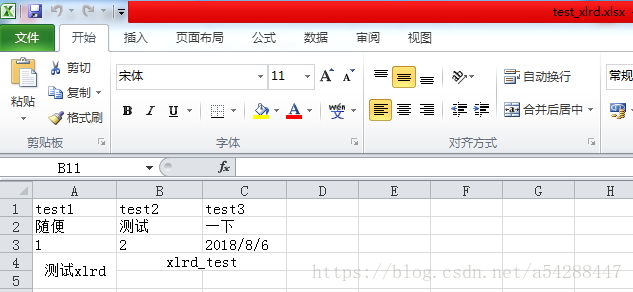``````# -*- coding: utf-8 -*-
import xlrd
#打开excel文件读取数据
exce = xlrd.open_workbook('C:/Users/ms/Desktop/test_xlrd.xlsx')

#获取excel中对应的sheet
print('所有sheet名称',exce.sheet_names()) #获取所有sheet名称
sheets = exce.sheets()  #获取所有sheets
#sheet = exce.sheets()    #也可以通过下标去访问某个具体的sheet
sheet1 = exce.sheet_by_name('Sheet1')    #通过sheet名称获取
#sheet2 = exce.sheet_by_index(1) #通过下标获取某个sheet

#获取sheet中行数和列数
nrows = sheet1.nrows
ncols = sheet1.ncols
print('对应sheet中行数：%d行，列数：%d列'% (nrows,ncols))

#获取sheet中整行或整列的数据(数组)
row1 = sheet1.row_values(3)     #通过下标获取某一行的数据
col1 = sheet1.col_values(0)     #通过下标获取某一列的数据
print('某行的数据：',row1)
print('某列的数据：',col1)

#获取sheet中某个单元格的数据
cell_A3 = sheet1.cell(2,0).value    #第三行第一列
cell_B2 = sheet1.cell(1,1).value    #第二行第二列
cell_C3 = sheet1.cell(2,2).value    #第三行第三列
print('第一列第三行：',cell_A3)
print('第二行第二列：',cell_B2)
print('第三行第三列：',cell_C3)

#获取单元格数据类型
A3_ctype = sheet1.cell(2,0).ctype   #数字类型
B2_ctype = sheet1.cell(1,1).ctype   #str类型
C3_ctype = sheet1.cell(2,2).ctype   #data类型
print('数字类型：',A3_ctype)
print('str类型：',B2_ctype)
print('data类型：',C3_ctype)
#ctype：0 empty,1 string, 2 number, 3 date, 4 boolean, 5 error``````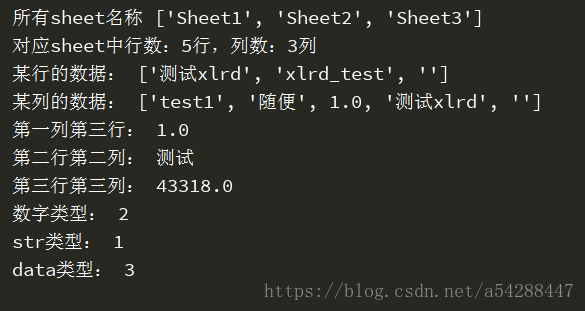``````# -*- coding: utf-8 -*-
import xlrd
exce = xlrd.open_workbook('C:/Users/ms/Desktop/test_xlrd.xlsx')
sheet1 = exce.sheet_by_name('Sheet1')
print(sheet1.cell(4,0).value)
print(sheet1.cell(3,0).value)
print(sheet1.cell(3,2).value)
print(sheet1.cell(3,1).value)``````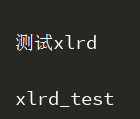``print(sheet1.merged_cells)``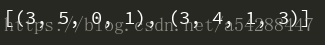``````# -*- coding: utf-8 -*-
import xlrd
exce = xlrd.open_workbook('C:/Users/ms/Desktop/test_xlrd.xlsx')
sheet1 = exce.sheet_by_name('Sheet1')
def row_col(sheet):
merge = []
for(row,row_range,col,col_range) in sheet.merged_cells:
merge.append([row,col])
for index in merge:
print(sheet.cell(index,index).value)
row_col(sheet1)``````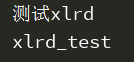``````# -*- coding: utf-8 -*-
import xlrd
exce = xlrd.open_workbook('C:/Users/ms/Desktop/test_xlrd.xlsx')
sheet1 = exce.sheet_by_name('Sheet1')

#处理单元格内容为data格式的数据
print(xlrd.xldate_as_datetime(sheet1.cell(2,2).value,0))    #转换成日期格式
print(xlrd.xldate_as_tuple(sheet1.cell(2,2).value,0))   #返回元组``````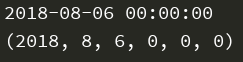``````#如果ctype等于3，就用时间格式处理
def xldate_datetime(sheet,row,col):
if(sheet.cell(row,col).ctype==3):
date_value = xlrd.xldate_as_datetime(sheet.cell(row,col).value,0)
return date_value
print(xldate_datetime(sheet1,2,2))``````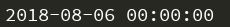o

### osc_1ee7cxmx#### 暂无文章

06/02
20
0

yiren081
34分钟前
21
0
Matlab系列之运算符和标点符号的功能介绍

07/06
18
0
Java源码系列（1）:Comparable和Comparator的区别

2018/09/19
25
0

55分钟前
14
0